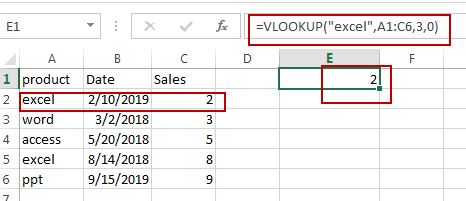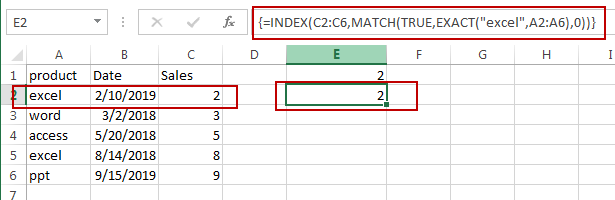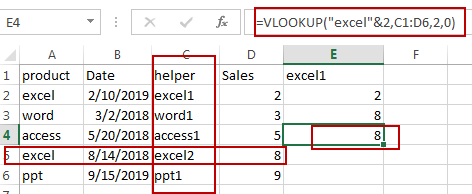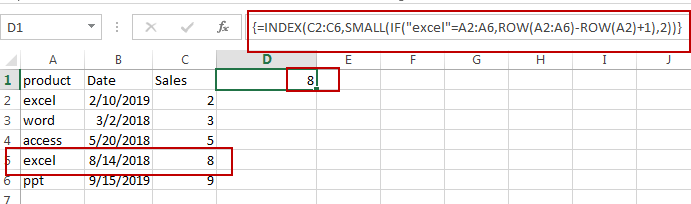# How to Get the First, 2nd or Nth Match Using Vlookup/Index/Match

This post will guide you how get the nth matching values with VLOOKUP function in Excel. How do I find the nth match value with Index/Match formula in Excel. How to find the first, second, third or nth matching value from a range of cells using VLOOKUP formula.

## Find the First Match Value Using VLOOKUP

Assuming that you have a list of data in range A1:C6, and you want to find a text string “excel” in this table, and then return its sales value. How to find the first match value in Excel. You can use a formula based on the VLOOKUP function to achieve the result of finding the first matching value in sales column. Like this:

`=VLOOKUP("excel",A1:C6,3,0)`

Type this formula in a blank cell and then press Enter key in your keyboard. This formula should be returned the first matching value.## Find the First Match Value Using Index/Match

You can also use another array formula based on the INDEX function in combination with the MATCH function to get the first match value. Just use the following formula:

`=INDEX(C2:C6,MATCH(TRUE,EXACT("excel",A2:A6),0))`

You need to type this formula into a blank cell and then press Ctrl +Alt +Enter keys to change it as array formula.## Find the Nth Match Value Using VLOOKUP

If you want to find the second or third or nth matching value in range A1:C6 using VLOOKUP function. And you need to create a helper column before the sales column firstly. Then type the following formula into Cell C2:

`=A2&COUNTIF(\$A\$2:\$A2,A2)`

This formula will create unique lookup values in helper column.

Then you need to use the following formula based on the VLOOKUP function to find the second matching value:

`=VLOOKUP("excel"&2,C1:D6,2,0)`Note: if you want to find the third matching values, just replace “excel”&2 with “excel”&3. And if you need to find the nth matching values, just change number 3 to number n.

## Find the Nth Match Value Using Index/Small

You can use another formula based on the INDEX function, The SMALL function, the IF function, and the ROW function to achieve the result of finding the Nth matching value. Just type the following array formula into a blank cell, and then press Ctrl +Alt + Enter keys.

`=INDEX(C2:C6,SMALL(IF("excel"=A2:A6,ROW(A2:A6)-ROW(A2)+1),2))`Video: Find 2nd, 3rd or Nth Match Value

### Related Functions

• Excel VLOOKUP function
The Excel VLOOKUP function lookup a value in the first column of the table and return the value in the same row based on index_num position.The syntax of the VLOOKUP function is as below:= VLOOKUP (lookup_value, table_array, column_index_num,[range_lookup])….
• Excel SMALL function
The Excel SMALL function returns the smallest numeric value from the numbers that you provided. Or returns the smallest value in the array.The syntax of the SMALL function is as below:=SMALL(array,nth) …
• Excel INDEX function
The Excel INDEX function returns a value from a table based on the index (row number and column number)The INDEX function is a build-in function in Microsoft Excel and it is categorized as a Lookup and Reference Function.The syntax of the INDEX function is as below:= INDEX (array, row_num,[column_num])…
• Excel ROW function
The Excel ROW function returns the row number of a cell reference.The ROW function is a build-in function in Microsoft Excel and it is categorized as a Lookup and Reference Function.The syntax of the ROW function is as below:= ROW ([reference])….
• Excel IF function
The Excel IF function perform a logical test to return one value if the condition is TRUE and return another value if the condition is FALSE. The IF function is a build-in function in Microsoft Excel and it is categorized as a Logical Function.The syntax of the IF function is as below:= IF (condition, [true_value], [false_value])….
• Excel COUNTIF function
The Excel COUNTIF function will count the number of cells in a range that meet a given criteria. This function can be used to count the different kinds of cells with number, date, text values, blank, non-blanks, or containing specific characters.etc.= COUNTIF (range, criteria)…
• Excel MATCH function
The Excel MATCH function search a value in an array and returns the position of that item.The syntax of the MATCH function is as below:= MATCH  (lookup_value, lookup_array, [match_type])….
• Excel EXACT function
The Excel EXACT function compares if two text strings are the same and returns TRUE if they are the same, Or, it will return FALSE.The syntax of the EXACT function is as below:= EXACT (text1,text2)…
Related Posts

Build Hyperlink With VLOOKUP in Excel

You might have come across a task in which you were assigned to build hyperlinks, which seems very easy, and if you are new to excel or don't have enough experience with it, then you might wonder about doing this ...

Calculate Total Cost with Excel VLOOKUP Function

In today's article we will show you how to calculate the total cost for a given weight using the Excel VLOOKUP function. This function will help us to find the appropriate unit price for that weight and then we can ...

Basic Price Discount Calculation with Excel VLOOKUP Function

We often encounter product discounts in our shopping. Depending on the level of spending, the mall will offer different percentages of discounts. Usually, the more you spend, the bigger the discount, while the less you spend, the smaller the discount. ...

Calculate Grades With VLookup in Excel

Why Should You Calculate Grades With VLookup Excel? If you're looking for a simple way to find out a student's grades, VLOOKUP Excel can do the trick. This function uses a lookup table to find the values and sort them ...

Convert State Names To Abbreviations

Assume that you got a task to convert the full state's name into the abbreviations in MS Excel, and for doing this task, you might choose to do it manually, which is an acceptable way only if you don't have ...

Find and Replace Multiple Values

This post will guide you how to find and replace multiple values at once with VBA macro or using formula  in Excel. How do I make multiple find and replace in Excel. Suppose that you have a few cells containing ...

Excel XLOOKUP Function

Excel XLOOKUP Function was added into Excel as a beta feature in August 2019 and is now accessible exclusively in Microsoft 365. (as of July 2021). However, if you fall into this category and often deal with big sets of ...

VLOOKUP Formula | Faster Trick with 2 VLOOKUPS

This post will guide you how to use 2 VLOOKUPS function to looking up data entries from a given range of cells in Microsoft Excel. VLOOKUP with 2 lookups can be faster than a single VLOOKUP in certain scenarios. The ...

VBA Macro For VLOOKUP From Another Sheet

In the previous post, you should know that how to fix or remove the #N/A error when using VLOOKUP formula to lookup value from another sheet. And this post will show you how to use VBA code to vlookup data ...

VLOOKUP From Another Sheet Not Working

In the previous post, you should know that how to fix or remove the #N/A error when using VLOOKUP formula to lookup value from another sheet. And this post will show you reasons why your VLOOKUP formula is not working ...

Sidebar